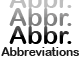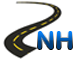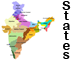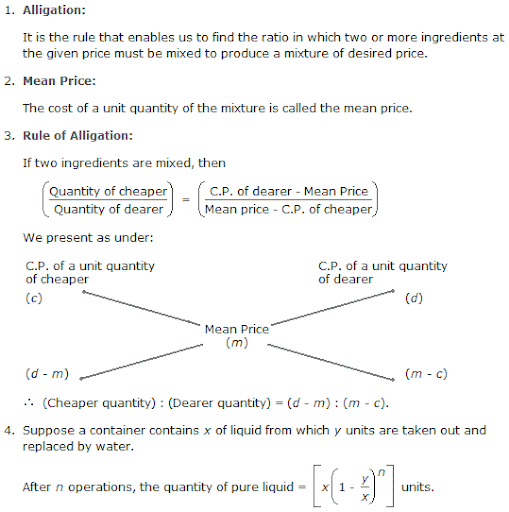Alligation or Mixture -List of AbbreviationsNational Highways in IndiaStates & Union Territories of IndiaCurrency of All Countries

# Alligation or Mixture

Overview:Sample Questions:

1. A Scan contains a mixture of two liquids A and B is the ratio 7 : 5. When 9 liters of mixture are drawn off and the can is filled with B, the ratio of A and B becomes 7 : 9. How many liters of liquid A was contained by the can initially?
A.     10    B.     20
C.     21     D.     25

Ans: C

2. In what ratio must a grocer mix two varieties of pulses costing Rs. 15 and Rs. 20 per kg respectively so as to get a mixture worth Rs. 16.50 kg?
A.     3 : 7    B.     5 : 7
C.    7 : 3     D.     7 : 5

Ans: C

3. How many kilogram of sugar costing Rs. 9 per kg must be mixed with 27 kg of sugar costing Rs. 7 per kg so that there may be a gain of 10% by selling the mixture at Rs. 9.24 per kg?
A.     36 kg    B.     42 kg
C.     54 kg    D.     63 kg

Ans: D

4. A container contains 40 liters of milk. From this container 4 liters of milk was taken out and replaced by water. This process was repeated further two times. How much milk is now contained by the container?
A.     26.34 liters B.     27.36 liters
C.     28 liters D.     29.l6 liters

Ans: D

5. In what ratio must a grocer mix two varieties of tea worth Rs. 60 a kg and Rs. 65 a kg so that by selling the mixture at Rs. 68.20 a kg he may gain 10%?
A.     3 : 2     B.     3 : 4
C.     3 : 5    D.     4 : 5

Ans: A

6. The cost of Type 1 rice is Rs. 15 per kg and Type 2 rice is Rs. 20 per kg. If both Type 1 and Type 2 are mixed in the ratio of 2 : 3, then the price per kg of the mixed variety of rice is:
A.     Rs. 18     B.     Rs. 18.50
C.     Rs. 19    D.     Rs. 19.50

Ans: A

7. In what ratio must rice at Rs.30 per kg mixed with rice at Rs.10.80 per kg so that the mixture be worth Rs.10per kg
A.     7:8 B.     6:7
C.     9:8 D.     8:7

Ans: D

8. In what ratio must wheat at Rs.3.20 per kg be mixed with wheat at Rs.2.90 per kg so that the mixture be worth Rs.3.08 per kg
A.     3:2 B.     6:8
C.     2:3 D.     5:3

Ans: A

9. How many kilograms of sugar costing Rs/.9 per kg must be mixed with 27kg of sugar costing Rs.7 per kg so that there may be gain of 10% by selling the mixture at Rs.9.24 per kg ?
A.     62     B.     63
C.     65    D.     64

Ans: B

10. In what ratio must water be mixed with milk to gain 16  2/3% on selling the mixture at cost price ?
A.     7:1 B.     1:7
C.     1:6 D.     2:7

Ans: C

11. Two vessels A and B contain milk and water mixed in the ratio 8:5 and 5:2 res. The ratio in which these two mixtures be mixed to get a new mixture containing milk and a water in the ratio  9:4?
A.     2:8 B.     3:7
C.     3:8 D.     2:7

Ans: D

12. In what ratio water be mixed with milk costing Rs.12 per liter to obtain a mixture worth of Rs.8 per liter?
A.     1:2 B.     2:1
C.     3:2 D.     2:3

Ans: A

13. A sum of Rs.4000 is lent out in two parts, one at 8% simple interest and the other at 10% simple interest. In the annual interest is Rs.352, the sum lent at 8% is
A.     Rs. 2400 B.     Rs. 2350
C.     Rs. 2650 D.     Rs. 2100

Ans: A

14. A merchant has 100kg of sugar, part of which he sells at 8% profit and the rest at 18% profit. He gains 14% on the whole. The quantity sold at 18% profit is
A.     600kg B.     Rs. 700kg
C.     645kg D.     Rs. 750kg

Ans: A

15. Two vessels A and B contain milk and water mixed in the ratio 4:3 and 2:3 in what ratio must these mixtures be mixed to form  new mixture containing half milk and half water?
A.     7:8 B.     6:7
C.     9:8 D.     7:5

Ans: D

16. A jar full of whisky contains 40% alcohol. A part of this whisky is replaced by another containing 19% alcohol and now the percentage of alcohol was found to be 26% the quantity of whisky replaced is
A.     3/4 B.     2/3
C.     2/5 D.     3/5

Ans: B

17. 729ml of mixture contains milk and water in the ratio 7:2 how much more wate is to be added to get a new mixture containing milk and water in the ratio 7:3?
A.     76ml B.     81ml
C.     79ml D.     84ml

Ans: B

18. A sum of Rs.312 was divided among 100boys and girls in such a way that the boy gets Rs.3.60 and each girl Rs.2.40 the number of girls is
A.     36 B.     40
C.     47 D.     44

Ans: B

19. A man covered a distance of 2000km in 18 hours partly by bus at 72kmph and partly by train at 16kmph the distance covered by bus is
A.     760ml B.     801ml
C.     720ml D.     840ml

Ans: C

20. A sum of rs.36.90 is made up of 180 coins which are either 10 paise coins or 25 p coins. The number of 10 p coins is
A.     53 B.     54
C.     55 D.     56

Ans: B

21. A dishonest milk man professes to sell his milk at cost price but he mixed it with water and thereby gains 25%. The percentage of water in the mixture is
A.     45% B.     21%
C.     20% D.     23%

Ans: C

22. A mixture of 20kg of spirit and water contains 10 water How much water must be added to this mixture to raise the percentage of water to 25%?
A.     4.2kg B.     5kg
C.     5.5kg D.     4kg

Ans 4kg

23. A container contains 40kg of milk, from this container 4kg of milk was taken out and replaced by water. This process was repeated further two times. How much milk is now contained by the container?
A.     27.94kg B.     29.16kg
C.     28.13kg D.     29.13kg

Ans: B

24. A can contains a mixture of two liquids A and B in the ratio 7:5 when 9 liters of mixture are drawn off and the can is filled with B, the ratio of A and B becomes 7:9 how many liters of liquids A was contained by the can initially?
A.     22 B.     21
C.     26 D.     23

Ans: B

25. In what ratio water be mixed with milk costing Rs.12 per liter to obtain a mixture worth of Rs.8 per liter?
A.     1:3 B.     3:1
C.     2:1 D.     1:2

Ans: DClick Here to Find Latest Jobs and Current Affairs

Updated: May 31, 2013 — 10:46 am